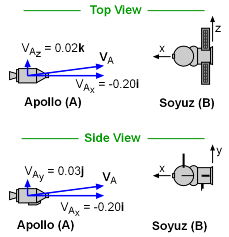Ch 4. Particle Momentum and Impulse Multimedia Engineering Dynamics Impulse & Momentum Consv. Linear Momentum Impact AngularMomentum MassFlow
 Chapter - Particle - 1. General Motion 2. Force & Accel. 3. Energy 4. Momentum - Rigid Body - 5. General Motion 6. Force & Accel. 7. Energy 8. Momentum 9. 3-D Motion 10. Vibrations Appendix Basic Math Units Basic Equations Sections Search eBooks Dynamics Fluids Math Mechanics Statics Thermodynamics Author(s): Kurt Gramoll ©Kurt GramollDYNAMICS - CASE STUDY IntroductionProblem Diagram On July 15, 1975, NASA and the Soviet space program set a new precedent at the height of the Cold War. NASA's Apollo CSM attempts to dock with the Soviet's Soyuz Space Station. What is known: The mass of the Apollo is mA = 18.0 Mg. The mass of the Soyuz is mB = 6.6 Mg. The Soyuz is stationary relative to the reference frame shown. The Apollo approaches relative to Soyus with the velocity vA = -0.20i + 0.03j + 0.02k m/s The force exerted by the docking collars is perpendicular to the longitudinal axis. In other words, the line of impact between the two space craft will be along the x-axis. Questions If the docking is successful, what is the velocity of the combined center of mass of the vehicles? If the docking is unsuccessful, and the coefficient of restitution is e = 0.95, what is the velocity of each spacecraft after the impact. Approach If the docking is successful, the conservation of linear momentum is sufficient to determine the velocity of the combined mass after the impact. If the docking is unsuccessful, the conservation of linear momentum, together with an equation involving the coefficient of restitution, can be used to determine the velocities of each ship after the impact

Practice Homework and Test problems now available in the 'Eng Dynamics' mobile app
Includes over 400 problems with complete detailed solutions.
Available now at the Google Play Store and Apple App Store.